## Frequency graphing, amplitude problems.

 So I'm testing this completely basic circuit out.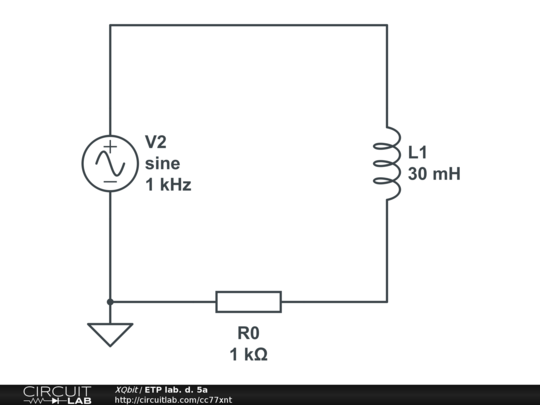When I run the frequency sweep from 50hz-100kHz although I've tried setting the amplitude in both the DC sweep (as just a single custom value of 5) and in the voltage source properties, no matter what, in the graph it shows that V(un2)-V(un3) (the voltage drop on the inductive coil) is nearing 1 Volt instead of 5 how logic dictates. Is this a bug, or have I made an obvious mistake which is not so obvious to me? by XQbit April 12, 2012 And I tried altering the amplitudes and it still shows the same graph, which seems to assume that the amplitude is 1 volt. Sorry for the double post, forgot to add it. by XQbit April 12, 2012 The voltage across the coil is going to have a DC and an AC component. The DC compionent is going to be proportional to the DC resistance of the coil. The AC component is going to be proportional to the AC reactance. I don't see why it should be 5 volts, AC or Dc. by arduinohacker April 12, 2012 A frequency domain response is usually independent of signal amplitudes. I think that when you do a frequency domain analysis, the signal source defaults to a fixed 1V and it ignores your amplitude setting as that is only used for the Time Domain analysis. That is how it works in SPICE and I'm assuming CircuitLab does much the same thing. The reason is because the AC analysis assumes that a frequency response analysis is to be carried out (to give a result such as in a Bode plot which is the gain or loss and phase of the circuit irrespective of input and output signal amplitudes) and assumes all components are linear and then carries out a small signal analysis (where all components are, by definition, linear). Frequency response plotting for non-linear circuits is possible but that is a very different game and not part of the standard SPICE analysis toolkit. If you do a time domain analysis you should find that the signals scale with your input. by signality April 12, 2012 @signality is not quite correct. In SPICE, one typically uses unity value AC sources, but an AC analysis can be done with other values of AC input, and the results will reflect this. The key here is that there are THREE voltages the simulator associates with the source: DC value (offset), (for DC and time domain) transient SINE amplitude (for time domain analysis), and AC value (for frequency domain analysis). Unlike SPICE, there seems no way in CircuitLab for the user to set that third value. Intuition would lead us to expect that the AC value and the transient amplitude are the same parameter, but the documentation clearly states that AC sources are set to unity magnitude: "CircuitLab makes this chosen input a sine wave of magnitude 1, and will sweep the frequency from the chosen start frequency to the end frequency in Hertz." This makes perfect sense when you view results in dB, but that convention is clearly confusing here. by CarlSawtell April 13, 2012 @CarlSawtell is right of course. For plots, such as gain, transimpedance or transconductance plots, where the value to be plotted is the ratio of output/input then clearly the ratio will be independent of input amplitude. Plotting just the output value should reflect the input amplitude. Sorry about any confusion that may have caused. One thing to beware of is that the DC value (offset) of a DC or waveform source such as a pulse or sine can affect the frequency domain analysis if there is a voltage or current dependent component in the signal path. An example of this is shown here: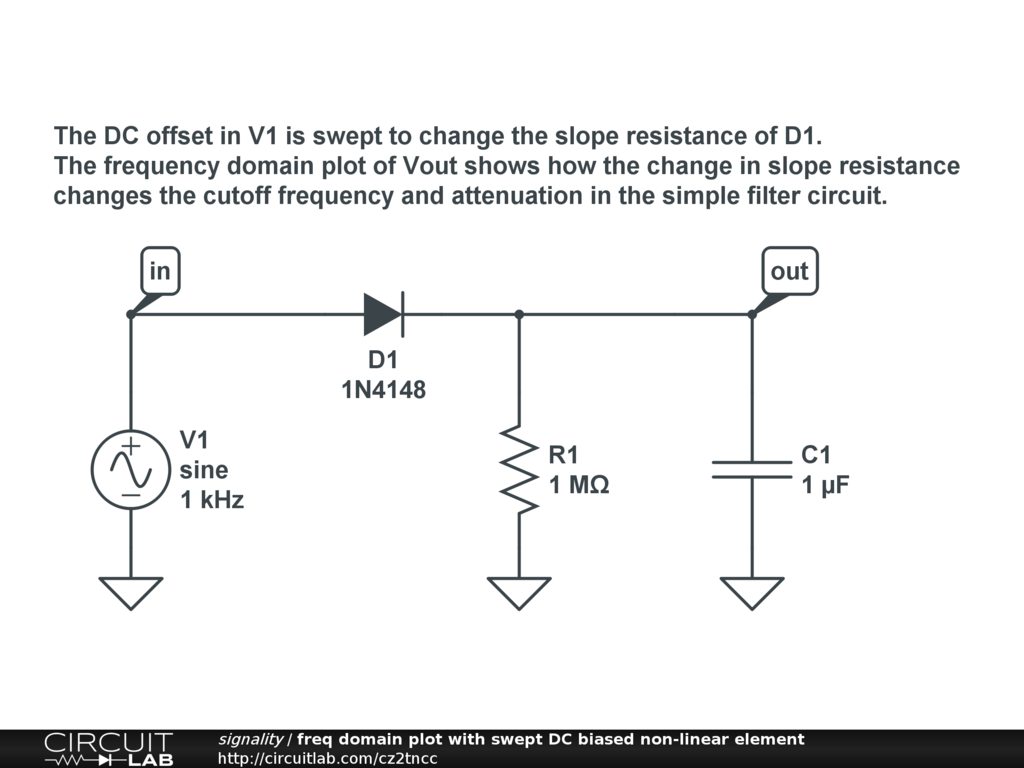by signality April 13, 2012 This is not good - what if I have a circuit with multiple AC sources of different amplitudes? by gbell12 August 25, 2012 @gbell12, this example shows how to do what you are asking about: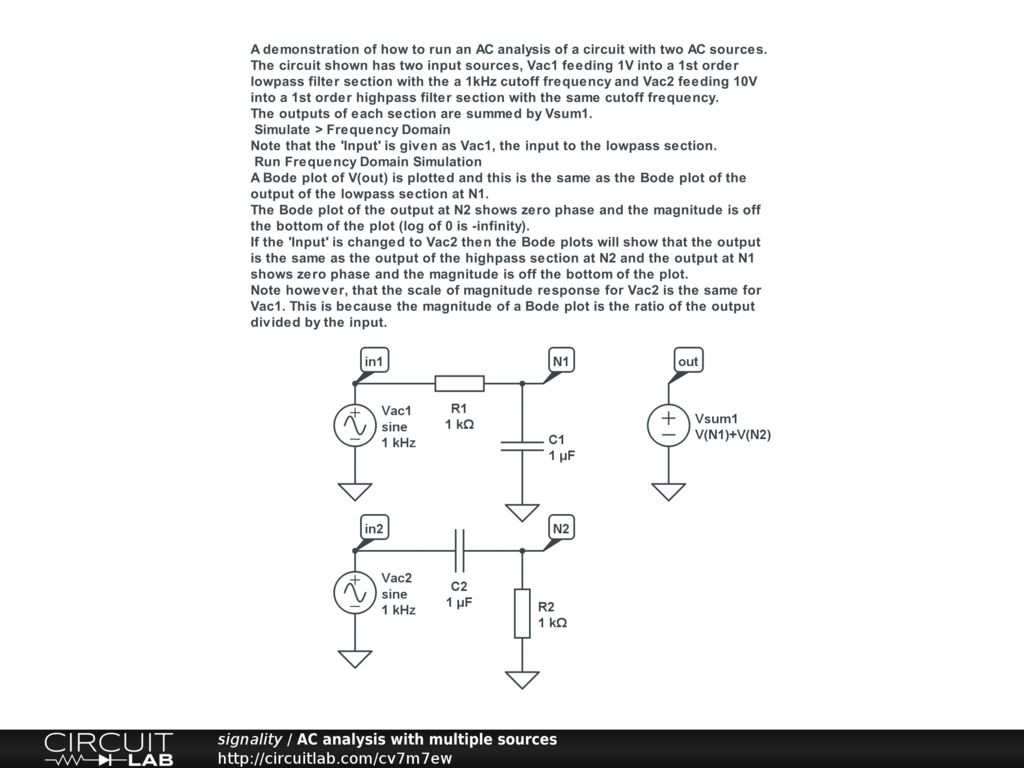by signality August 25, 2012 Thanks for that @signality. Unfortunately, it highlights the same limitation with CircuitLab as discussed above. Namely, that even though you've set the source to an amplitude of 10 V, the output is only 1 V. If I want to accurately simulate an AC circuit with multiple sources, CircuitLab needs to respect the amplitudes set on the sources. Moreover, I think the tool needs the option of sweeping the frequency of more than one source at once. by gbell12 August 28, 2012 You've misunderstood the majority of my points. You absolutely do need AC amplitude of sources to get correct AC amplitude of voltages or currents at various points in the circuit. When I say "sweeping the frequency of multiple sources", I mean one frequency set on multiple sources. Circuitlab makes you pick an AC source to sweep. QUCS sweeps all of them. I can use QUCS to find out any current or voltage at any point in a complicated, multiple-source AC circuit using their AC analysis. If I'm interested in the circuit's voltages and currents at 1 kHz, I set the sweep to 1 kHz - 2 kHz with 2 steps, and I get the circuit's steady-state currents and voltages at two frequencies... 1 kHz and 2 kHz (I haven't figured out how to get just one frequency, so I set it up to sweep at two and just ignore the second). I cannot do this with CircuitLab for two reasons: 1) CircuitLab does not use the amplitudes of the AC sources in its AC analysis. 2) CircuitLab will set the frequency of just one source. Maybe that's OK when I'm only interested in one frequency. I haven't investigated further since point 1) is a showstopper. by gbell12 August 29, 2012 Sorry if I misunderstand you. Even so, I still don't understand quite what you mean. Could you post a link to a QUCS example or draw something in CL just to show (even if CL won't simulate quite what you want)? by signality August 29, 2012 The original post that started this thread had an example. What's the voltage across L1 if V2 is 240 Vp? by gbell12 August 29, 2012 I take your point about be unable to directly plot - in CL - the voltage across L1 for a given V2 in the frequency domain. It would be convenient to be able to plot this directly compared to having to take the magnitude given by the Bode plot and to then multiply it by the amplitude of the AC source. However, for a fixed or even a swept range of frequency, you can just as easily do that using a time domain simulation. OK this may be beyond the current scope of CL but in a realistic simulation this may be a more reliable option because it would take account of any non-linearities such as inductor saturation effects which an AC simulation would ignore. The part of your concern that still escapes me is when you would want to be able to do an analysis with two AC sources swept at the same time. If they are the same frequency then why not just use one source connected to both inputs? If they are different frequencies then how do you display and interpret the results? One case I can think of where you might want to put the same frequency into two inputs and sweep both together is if the two inputs differ in phase. For instance if you want to look at the response of some sort of quadrature network where you have two inputs at the same frequency but with 90 degrees difference between them. Examples of such networks can be found in SSB modulation and demodulation and in audio pitch shifters. An example of the sort of multi-source AC sweep would be good to help illustrate this point. :) by signality September 01, 2012 Any basic AC circuit is beyond CL, Signality.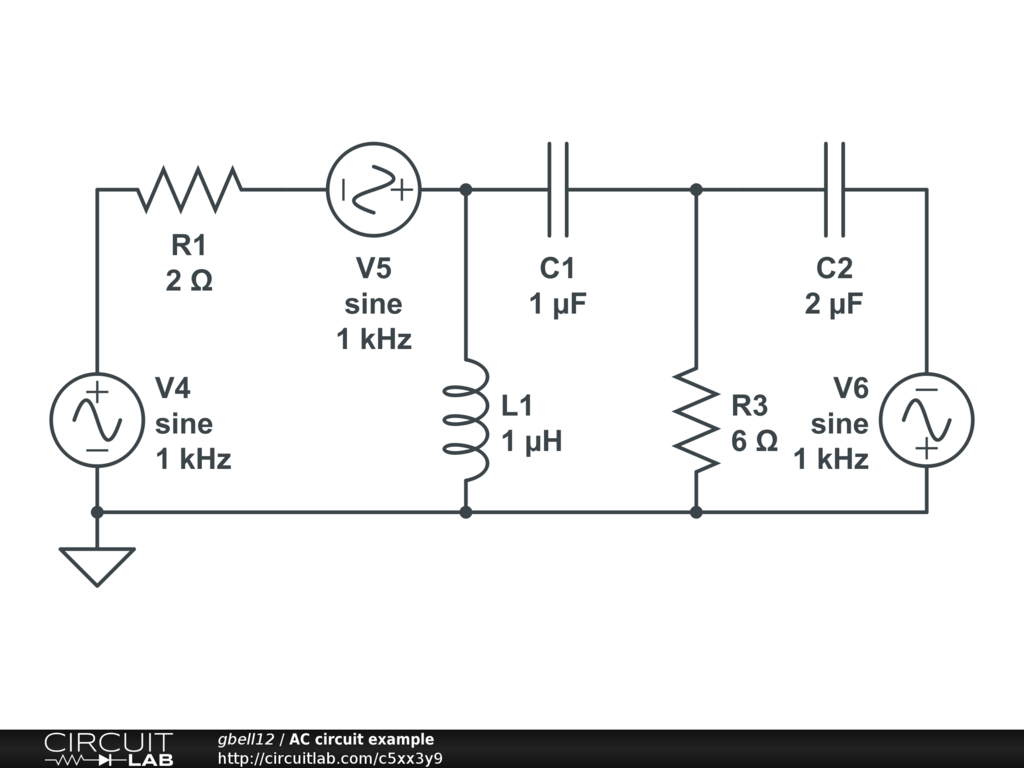V4 is 120 Vp, V5 is 110 Vp, V6 is 232 Vp. What's the voltage across C1 when every source is 50 Hz? by gbell12 September 02, 2012 It is not impossible to do in CL but I agree that if you need to do this sort of AC analysis then CL forces you to jump through some extra hoops compared to other simulators. A workaround is to use controlled sources like this: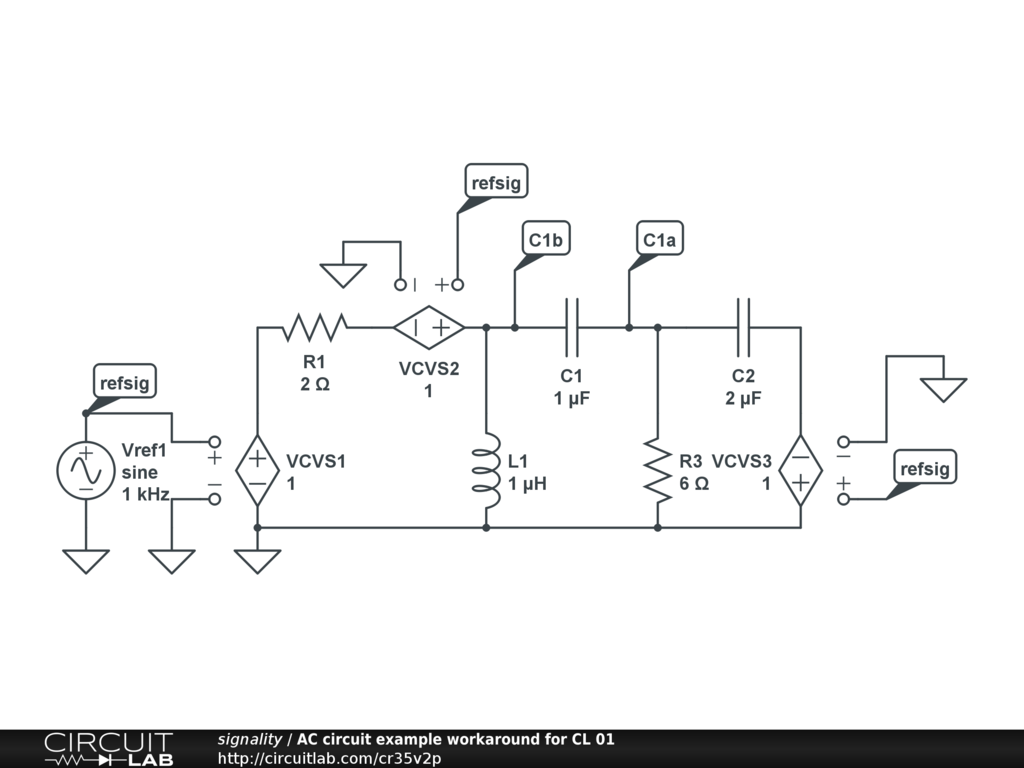This issue probably needs to be raised as a feature request. by signality September 03, 2012 And here we are, finally, back where we started, at the original post at the top of this thread, which laments that AC analysis in CL assumes a 1 Vp signal. This is not good. by gbell12 September 03, 2012 Rather than just saying that other simulators do something and CL does not therefore CL is bad, it is useful to explore what features might be required and some examples of why they might be desirable as well as possible workarounds before adding to the pile of feature requests that the CL authors are steadily working through. I have now posted a suitable feature request: https://www.circuitlab.com/forums/feature-requests/topic/cs5774ts/signal-amplitudes-with-multiple-ac-sources-rather-than-gain/ by signality September 03, 2012 And it's not as simple as I thought ... See: https://www.circuitlab.com/forums/modeling-and-simulation/topic/vsuz86e2/another-question-about-ac-analysis-with-multiple-sources/ by signality November 19, 2012